## Example Questions

2 Next →

### Example Question #251 : Geometry

In the following diagram, linesandare parallel to each other. What is the value for?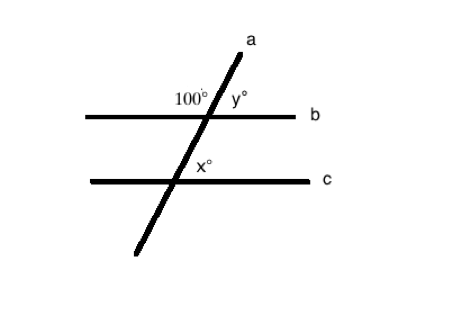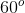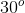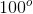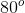It cannot be determinedExplanation:

When two parallel lines are intersected by another line, the sum of the measures of the interior angles on the same side of the line is 180°. Therefore, the sum of the angle that is labeled as 100° and angle y is 180°. As a result, angle y is 80°.

Another property of two parallel lines that are intersected by a third line is that the corresponding angles are congruent. So, the measurement of angle x is equal to the measurement of angle y, which is 80°.

### Example Question #1 : Sat Mathematics

The measure of the supplement of angle A is 40 degrees larger than twice the measure of the complement of angle A. What is the sum, in degrees, of the measures of the supplement and complement of angle A?

90

50

140

40

190

190

Explanation:

Let A represent the measure, in degrees, of angle A. By definition, the sum of the measures of A and its complement is 90 degrees. We can write the following equation to determine an expression for the measure of the complement of angle A.

A + measure of complement of A = 90

Subtract A from both sides.

measure of complement of A = 90 – A

Similarly, because the sum of the measures of angle A and its supplement is 180 degrees, we can represent the measure of the supplement of A as 180 – A.

The problem states that the measure of the supplement of A is 40 degrees larger than twice the measure of the complement of A. We can write this as 2(90-A) + 40.

Next, we must set the two expressions 180 – A and 2(90 – A) + 40 equal to one another and solve for A:

180 – A = 2(90 – A) + 40

Distribute the 2:

180 - A = 180 – 2A + 40

180 + A = 180 + 40

Subtract 180 from both sides:

A = 40

Therefore the measure of angle A is 40 degrees.

The question asks us to find the sum of the measures of the supplement and complement of A. The measure of the supplement of A is 180 – A = 180 – 40 = 140 degrees. Similarly, the measure of the complement of A is 90 – 40 = 50 degrees.

The sum of these two is 140 + 50 = 190 degrees.

### Example Question #11 : How To Find An Angle Of A Line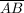is a straight line.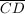intersectsat point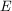. If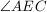measures 120 degrees, what must be the measure of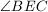?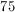degrees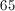degrees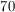degrees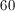degreesdegrees

Explanation:must add up to 180 degrees. So, ifis 120,(the supplementary angle) must equal 60, for a total of 180.

### Example Question #11 : Geometry

Two parallel lines are intersected by a transversal. If the minor angle of intersection between the first parallel line and the transversal is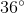, what is the minor angle of intersection between the second parallel line and the transversal?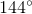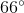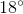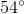Explanation:

When a line intersects two parallel lines as a transversal, it always passes through both at identical angles (regardless of distance or length of arc).

### Example Question #11 : Plane Geometry

Angle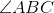measures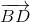is the bisector of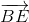is the bisector ofWhat is the measure of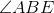?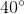Explanation:Let's begin by observing the larger angle.is cut into two 10-degree angles by. This means that anglesand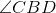equal 10 degrees. Next, we are told thatbisects, which creates two 5-degree angles.consists of, which is 10 degrees, and, which is 5 degrees. We need to add the two angles together to solve the problem.### Example Question #2062 : Hspt Mathematics

If,, and, what is the measure, in degrees, of32

122

58

148

62

148

Explanation:

The question states that. The alternate interior angle theorem states that if two parallel lines are cut by a transversal, then pairs of alternate interior angles are congruent; therefore, we know the following measure:The sum of angles of a triangle is equal to 180 degrees. The question states that; therefore we know the following measure:Use this information to solve for the missing angle:The degree measure of a straight line is 180 degrees; therefore, we can write the following equation:The measure ofis 148 degrees.

### Example Question #2 : How To Find An Angle Of A Line

Lines A and B in the diagram below are parallel. The triangle at the bottom of the figure is an isosceles triangle.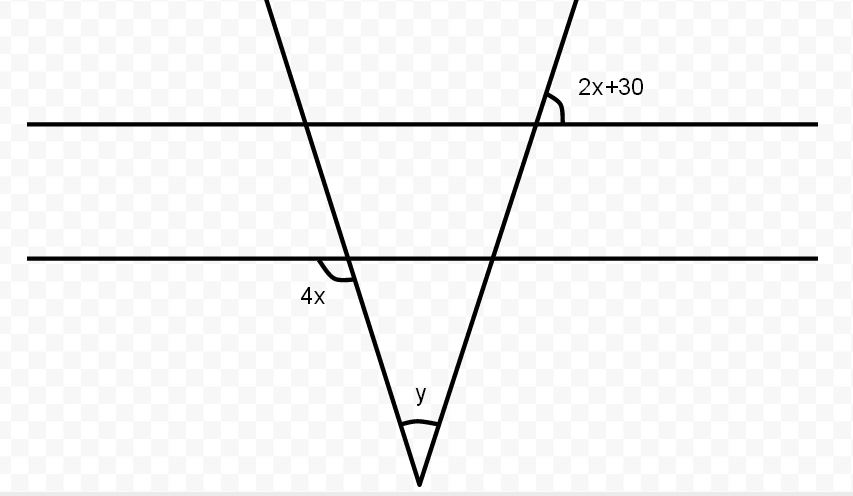What is the degree measure of angle?Explanation:

Since A and B are parallel, and the triangle is isosceles, we can use the supplementary rule for the two angles,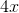and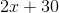which will sum up to. Setting up an algebraic equation for this, we get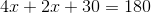. Solving for, we get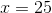. With this, we can get either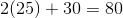(for the smaller angle) or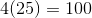(for the larger angle - must then use supplementary rule again for inner smaller angle). Either way, we find that the inner angles at the top are 80 degrees each. Since the sum of the angles within a triangle must equal 180, we can set up the equation as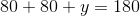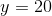degrees.

2 Next →

### All ACT Math Resources